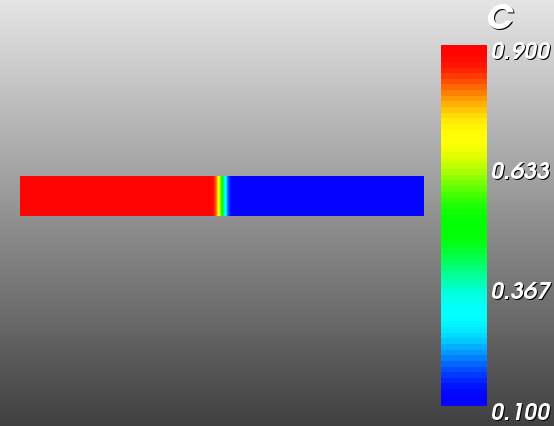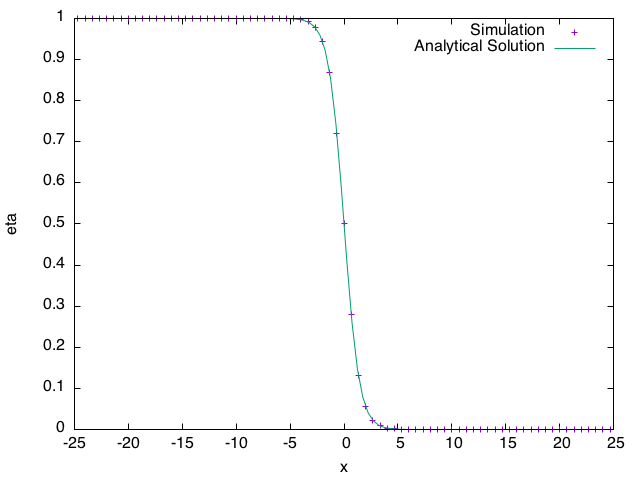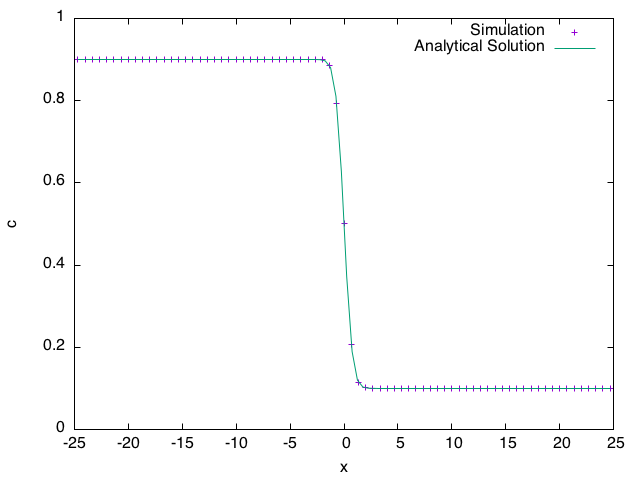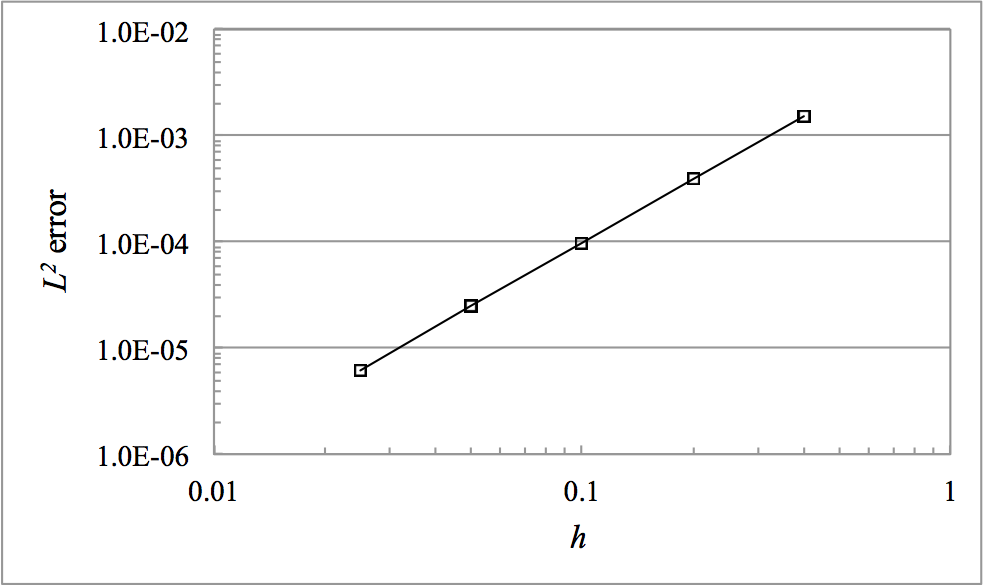# Analytical Solution for a 1D equilibrium interfaceComposition for 2D simulation domain.Order parameter along .Composition along .

In the KKS model, an analytical solution exists for the order parameter and composition through a 1D equilibrium interface:

(1)

(2)

where we use the switching function , the gradient energy coefficient and the barrier function height . (Note that there is a typo in Eq. (49) of Kim et al. (1999), should be in the denominator of the argument to the function, not .) In the following example, we equilibrate a flat interface between a solid phase (, , ) and a liquid phase (, , ) in a 2D simulation. The vector postprocessor LineValueSampler is used to obtain the values of and along , the results are output to a CSV file, and plotted together with the 1D analytical solution. (We will use no-flux boundary conditions, so a boundary conditions block is not required in the input file.)

#
# KKS simple example in the split form
#

[Mesh]
type = GeneratedMesh
dim = 2
nx = 150
ny = 15
nz = 0
xmin = -25
xmax = 25
ymin = -2.5
ymax = 2.5
zmin = 0
zmax = 0
[]

[AuxVariables]
[./Fglobal]
order = CONSTANT
family = MONOMIAL
[../]
[]

[Variables]
# order parameter
[./eta]
order = FIRST
family = LAGRANGE
[../]

# solute concentration
[./c]
order = FIRST
family = LAGRANGE
[../]

# chemical potential
[./w]
order = FIRST
family = LAGRANGE
[../]

# Liquid phase solute concentration
[./cl]
order = FIRST
family = LAGRANGE
initial_condition = 0.1
[../]
# Solid phase solute concentration
[./cs]
order = FIRST
family = LAGRANGE
initial_condition = 0.9
[../]
[]

[Functions]
[./ic_func_eta]
type = ParsedFunction
value = '0.5*(1.0-tanh((x)/sqrt(2.0)))'
[../]
[./ic_func_c]
type = ParsedFunction
value = '0.9*(0.5*(1.0-tanh(x/sqrt(2.0))))^3*(6*(0.5*(1.0-tanh(x/sqrt(2.0))))^2-15*(0.5*(1.0-tanh(x/sqrt(2.0))))+10)+0.1*(1-(0.5*(1.0-tanh(x/sqrt(2.0))))^3*(6*(0.5*(1.0-tanh(x/sqrt(2.0))))^2-15*(0.5*(1.0-tanh(x/sqrt(2.0))))+10))'
[../]
[]

[ICs]
[./eta]
variable = eta
type = FunctionIC
function = ic_func_eta
[../]
[./c]
variable = c
type = FunctionIC
function = ic_func_c
[../]
[]

[Materials]
# Free energy of the liquid
[./fl]
type = DerivativeParsedMaterial
f_name = fl
args = 'cl'
function = '(0.1-cl)^2'
[../]

# Free energy of the solid
[./fs]
type = DerivativeParsedMaterial
f_name = fs
args = 'cs'
function = '(0.9-cs)^2'
[../]

# h(eta)
[./h_eta]
type = SwitchingFunctionMaterial
h_order = HIGH
eta = eta
[../]

# g(eta)
[./g_eta]
type = BarrierFunctionMaterial
g_order = SIMPLE
eta = eta
[../]

# constant properties
[./constants]
type = GenericConstantMaterial
prop_names  = 'M   L   eps_sq'
prop_values = '0.7 0.7 1.0  '
[../]
[]

[Kernels]
active = 'PhaseConc ChemPotSolute CHBulk ACBulkF ACBulkC ACInterface dcdt detadt ckernel'

# enforce c = (1-h(eta))*cl + h(eta)*cs
[./PhaseConc]
type = KKSPhaseConcentration
ca       = cl
variable = cs
c        = c
eta      = eta
[../]

# enforce pointwise equality of chemical potentials
[./ChemPotSolute]
type = KKSPhaseChemicalPotential
variable = cl
cb       = cs
fa_name  = fl
fb_name  = fs
[../]

#
# Cahn-Hilliard Equation
#
[./CHBulk]
type = KKSSplitCHCRes
variable = c
ca       = cl
cb       = cs
fa_name  = fl
fb_name  = fs
w        = w
[../]

[./dcdt]
type = CoupledTimeDerivative
variable = w
v = c
[../]
[./ckernel]
type = SplitCHWRes
mob_name = M
variable = w
[../]

#
# Allen-Cahn Equation
#
[./ACBulkF]
type = KKSACBulkF
variable = eta
fa_name  = fl
fb_name  = fs
w        = 1.0
args = 'cl cs'
[../]
[./ACBulkC]
type = KKSACBulkC
variable = eta
ca       = cl
cb       = cs
fa_name  = fl
fb_name  = fs
[../]
[./ACInterface]
type = ACInterface
variable = eta
kappa_name = eps_sq
[../]
type = TimeDerivative
variable = eta
[../]
[]

[AuxKernels]
[./GlobalFreeEnergy]
variable = Fglobal
type = KKSGlobalFreeEnergy
fa_name = fl
fb_name = fs
w = 1.0
[../]
[]

[Executioner]
type = Transient
solve_type = 'PJFNK'

petsc_options_iname = '-pc_type -sub_pc_type -sub_pc_factor_shift_type'
petsc_options_value = 'asm      ilu          nonzero'

l_max_its = 100
nl_max_its = 100

num_steps = 50
dt = 0.1
[]

#
# Precondition using handcoded off-diagonal terms
#
[Preconditioning]
[./full]
type = SMP
full = true
[../]
[]

[VectorPostprocessors]
[./c]
type =  LineValueSampler
start_point = '-25 0 0'
end_point = '25 0 0'
variable = c
num_points = 151
sort_by =  id
execute_on = timestep_end
[../]
[./eta]
type =  LineValueSampler
start_point = '-25 0 0'
end_point = '25 0 0'
variable = eta
num_points = 151
sort_by =  id
execute_on = timestep_end
[../]

[]

[Outputs]
exodus = true
[./csv]
type = CSV
execute_on = final
[../]
[]

(modules/phase_field/examples/kim-kim-suzuki/kks_example_noflux.i)

# Verification against analytical solutionerror for order parameter .

To perform a more quantitative comparison of the simulation results to the analytical solution, we will calculate the norm of the difference between the simulation result and the analytical solution. The norm is defined for this case as

(3) where is the analytical solution, is the equilibrium solution from simulation, and is the domain. The norm can be obtained in the MOOSE framework using the ElementL2Error postprocessor. It can be shown from the properties of the finite element method that for the linear Lagrange elements used in the split formulation,

(4) where is the characteristic element size (for 2D square elements, is the length of one side) and is a constant specific to the problem. Thus, as the mesh is refined, error should decrease (at least) quadratically with .

In performing this comparison between the analytical solution and simulation results, if a no-flux boundary condition is used in the simulation, the order parameter and composition profiles may shift slightly in the or direction, even if the analytical solution is used as an initial condition. This makes it difficult to compare to an analytical solution centered at . Therefore, we simulate the half of the domain and use a Dirichlet boundary condition of and at , which prevents the interface from moving. For the verification, the size of the domain was also reduced to lower the computational cost.

To verify that error decreases quadratically with , see the plot showing error versus for in this problem. As expected, on a log-log scale, the points are fit well by a straight line. The slope was determined to be 1.995, in good agreement with the expected value of 2.

#
# KKS simple example in the split form
#

[Mesh]
type = GeneratedMesh
dim = 2
nx = 50
ny = 2
nz = 0
xmin = 0
xmax = 20
ymin = 0
ymax = 0.4
zmin = 0
zmax = 0
[]

[AuxVariables]
[./Fglobal]
order = CONSTANT
family = MONOMIAL
[../]
[]

[Variables]
# order parameter
[./eta]
order = FIRST
family = LAGRANGE
[../]

# hydrogen concentration
[./c]
order = FIRST
family = LAGRANGE
[../]

# chemical potential
[./w]
order = FIRST
family = LAGRANGE
[../]

# Liquid phase solute concentration
[./cl]
order = FIRST
family = LAGRANGE
initial_condition = 0.1
[../]
# Solid phase solute concentration
[./cs]
order = FIRST
family = LAGRANGE
initial_condition = 0.9
[../]
[]

[Functions]
[./ic_func_eta]
type = ParsedFunction
value = 0.5*(1.0-tanh((x)/sqrt(2.0)))
[../]
[./ic_func_c]
type = ParsedFunction
value = '0.9*(0.5*(1.0-tanh(x/sqrt(2.0))))^3*(6*(0.5*(1.0-tanh(x/sqrt(2.0))))^2-15*(0.5*(1.0-tanh(x/sqrt(2.0))))+10)+0.1*(1-(0.5*(1.0-tanh(x/sqrt(2.0))))^3*(6*(0.5*(1.0-tanh(x/sqrt(2.0))))^2-15*(0.5*(1.0-tanh(x/sqrt(2.0))))+10))'
[../]
[]

[ICs]
[./eta]
variable = eta
type = FunctionIC
function = ic_func_eta
[../]
[./c]
variable = c
type = FunctionIC
function = ic_func_c
[../]
[]

[BCs]
[./left_c]
type = DirichletBC
variable = 'c'
boundary = 'left'
value = 0.5
[../]
[./left_eta]
type = DirichletBC
variable = 'eta'
boundary = 'left'
value = 0.5
[../]
[]

[Materials]
# Free energy of the liquid
[./fl]
type = DerivativeParsedMaterial
f_name = fl
args = 'cl'
function = '(0.1-cl)^2'
[../]

# Free energy of the solid
[./fs]
type = DerivativeParsedMaterial
f_name = fs
args = 'cs'
function = '(0.9-cs)^2'
[../]

# h(eta)
[./h_eta]
type = SwitchingFunctionMaterial
h_order = HIGH
eta = eta
[../]

# g(eta)
[./g_eta]
type = BarrierFunctionMaterial
g_order = SIMPLE
eta = eta
[../]

# constant properties
[./constants]
type = GenericConstantMaterial
prop_names  = 'M   L   eps_sq'
prop_values = '0.7 0.7 1.0  '
[../]
[]

[Kernels]
# enforce c = (1-h(eta))*cl + h(eta)*cs
[./PhaseConc]
type = KKSPhaseConcentration
ca       = cl
variable = cs
c        = c
eta      = eta
[../]

# enforce pointwise equality of chemical potentials
[./ChemPotSolute]
type = KKSPhaseChemicalPotential
variable = cl
cb       = cs
fa_name  = fl
fb_name  = fs
[../]

#
# Cahn-Hilliard Equation
#
[./CHBulk]
type = KKSSplitCHCRes
variable = c
ca       = cl
cb       = cs
fa_name  = fl
fb_name  = fs
w        = w
[../]

[./dcdt]
type = CoupledTimeDerivative
variable = w
v = c
[../]
[./ckernel]
type = SplitCHWRes
mob_name = M
variable = w
[../]

#
# Allen-Cahn Equation
#
[./ACBulkF]
type = KKSACBulkF
variable = eta
fa_name  = fl
fb_name  = fs
w        = 1.0
args = 'cl cs'
[../]
[./ACBulkC]
type = KKSACBulkC
variable = eta
ca       = cl
cb       = cs
fa_name  = fl
fb_name  = fs
[../]
[./ACInterface]
type = ACInterface
variable = eta
kappa_name = eps_sq
[../]
type = TimeDerivative
variable = eta
[../]
[]

[AuxKernels]
[./GlobalFreeEnergy]
variable = Fglobal
type = KKSGlobalFreeEnergy
fa_name = fl
fb_name = fs
w = 1.0
[../]
[]

[Executioner]
type = Transient
solve_type = 'PJFNK'

petsc_options_iname = '-pc_type -sub_pc_type -sub_pc_factor_shift_type'
petsc_options_value = 'asm      ilu          nonzero'

l_max_its = 100
nl_max_its = 100
nl_abs_tol = 1e-10

end_time = 800
dt = 4.0
[]

#
# Precondition using handcoded off-diagonal terms
#
[Preconditioning]
[./full]
type = SMP
full = true
[../]
[]

[Postprocessors]
[./dofs]
type = NumDOFs
[../]
[./integral]
type = ElementL2Error
variable = eta
function = ic_func_eta
[../]
[]

[Outputs]
exodus = true
console = true
gnuplot = true
[]

(modules/phase_field/examples/kim-kim-suzuki/kks_example_dirichlet.i)
1. Seong Gyoon Kim, Won Tae Kim, and Toshio Suzuki. Phase-field model for binary alloys. Physical Review E, 60(6):7186–7197, December 1999. URL: http://link.aps.org/doi/10.1103/PhysRevE.60.7186, doi:10.1103/PhysRevE.60.7186.[BibTeX]